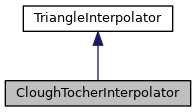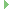CloughTocherInterpolator Class Reference

This is an implementation of a Clough-Tocher interpolator based on a triangular tessellation. More...

`#include <CloughTocherInterpolator.h>`

Inheritance diagram for CloughTocherInterpolator:[legend]

## Public Member Functions

CloughTocherInterpolator ()=default
Standard constructor. More...

CloughTocherInterpolator (NormVecDecorator *tin)
Constructor with a pointer to the triangulation as argument. More...

bool calcNormVec (double x, double y, QgsPoint &result) override
Calculates the normal vector and assigns it to vec (not implemented at the moment) More...

bool calcPoint (double x, double y, QgsPoint &result) override
Performs a linear interpolation in a triangle and assigns the x-,y- and z-coordinates to point. More...

virtual void setTriangulation (NormVecDecorator *tin)Public Member Functions inherited from TriangleInterpolator
virtual ~TriangleInterpolator ()=default

virtual bool calcNormVec (double x, double y, QgsPoint &result)=0
Calculates the normal vector and assigns it to vec. More...

virtual bool calcPoint (double x, double y, QgsPoint &result)=0
Performs a linear interpolation in a triangle and assigns the x-,y- and z-coordinates to point. More...

## Protected Member Functions

double calcBernsteinPoly (int n, int i, int j, int k, double u, double v, double w)
Calculates the Bernsteinpolynomials to calculate the Beziertriangle. 'n' is three in the cubical case, 'i', 'j', 'k' are the indices of the controllpoint and 'u', 'v', 'w' are the barycentric coordinates of the point. More...

void init (double x, double y)
Finds out, in which triangle the point with the coordinates x and y is. More...

## Protected Attributes

QgsPoint cp1 = QgsPoint( 0, 0, 0 )
Control point 1. More...

QgsPoint cp10 = QgsPoint( 0, 0, 0 )
Control point 10. More...

QgsPoint cp11 = QgsPoint( 0, 0, 0 )
Control point 11. More...

QgsPoint cp12 = QgsPoint( 0, 0, 0 )
Control point 12. More...

QgsPoint cp13 = QgsPoint( 0, 0, 0 )
Control point 13. More...

QgsPoint cp14 = QgsPoint( 0, 0, 0 )
Control point 14. More...

QgsPoint cp15 = QgsPoint( 0, 0, 0 )
Control point 15. More...

QgsPoint cp16 = QgsPoint( 0, 0, 0 )
Control point 16. More...

QgsPoint cp2 = QgsPoint( 0, 0, 0 )
Control point 2. More...

QgsPoint cp3 = QgsPoint( 0, 0, 0 )
Control point 3. More...

QgsPoint cp4 = QgsPoint( 0, 0, 0 )
Control point 4. More...

QgsPoint cp5 = QgsPoint( 0, 0, 0 )
Control point 5. More...

QgsPoint cp6 = QgsPoint( 0, 0, 0 )
Control point 6. More...

QgsPoint cp7 = QgsPoint( 0, 0, 0 )
Control point 7. More...

QgsPoint cp8 = QgsPoint( 0, 0, 0 )
Control point 8. More...

QgsPoint cp9 = QgsPoint( 0, 0, 0 )
Control point 9. More...

double der1X = 0.0
Derivative in x-direction at point1. More...

double der1Y = 0.0
Derivative in y-direction at point1. More...

double der2X = 0.0
Derivative in x-direction at point2. More...

double der2Y = 0.0
Derivative in y-direction at point2. More...

double der3X = 0.0
Derivative in x-direction at point3. More...

double der3Y = 0.0
Derivative in y-direction at point3. More...

QgsPoint lpoint1 = QgsPoint( 0, 0, 0 )
Stores point1 of the last run. More...

QgsPoint lpoint2 = QgsPoint( 0, 0, 0 )
Stores point2 of the last run. More...

QgsPoint lpoint3 = QgsPoint( 0, 0, 0 )
Stores point3 of the last run. More...

double mEdgeTolerance = 0.00001
Tolerance of the barycentric coordinates at the borders of the triangles (to prevent errors because of very small negative baricentric coordinates) More...

NormVecDecoratormTIN = nullptr
Association with a triangulation object. More...

QgsPoint point1 = QgsPoint( 0, 0, 0 )
First point of the triangle in x-,y-,z-coordinates. More...

QgsPoint point2 = QgsPoint( 0, 0, 0 )
Second point of the triangle in x-,y-,z-coordinates. More...

QgsPoint point3 = QgsPoint( 0, 0, 0 )
Third point of the triangle in x-,y-,z-coordinates. More...

## Detailed Description

This is an implementation of a Clough-Tocher interpolator based on a triangular tessellation.

The derivatives orthogonal to the boundary curves are interpolated linearly along a triangle edge.

Note
Not available in Python bindings

Definition at line 35 of file CloughTocherInterpolator.h.

## ◆ CloughTocherInterpolator() [1/2]

 CloughTocherInterpolator::CloughTocherInterpolator ( )
default

Standard constructor.

## ◆ CloughTocherInterpolator() [2/2]

 CloughTocherInterpolator::CloughTocherInterpolator ( NormVecDecorator * tin )

Constructor with a pointer to the triangulation as argument.

Definition at line 22 of file CloughTocherInterpolator.cpp.

## ◆ calcBernsteinPoly()

 double CloughTocherInterpolator::calcBernsteinPoly ( int n, int i, int j, int k, double u, double v, double w )
protected

Calculates the Bernsteinpolynomials to calculate the Beziertriangle. 'n' is three in the cubical case, 'i', 'j', 'k' are the indices of the controllpoint and 'u', 'v', 'w' are the barycentric coordinates of the point.

Definition at line 33 of file CloughTocherInterpolator.cpp.

## ◆ calcNormVec()

 bool CloughTocherInterpolator::calcNormVec ( double x, double y, QgsPoint & result )
overridevirtual

Calculates the normal vector and assigns it to vec (not implemented at the moment)

Implements TriangleInterpolator.

Definition at line 56 of file CloughTocherInterpolator.cpp.

## ◆ calcPoint()

 bool CloughTocherInterpolator::calcPoint ( double x, double y, QgsPoint & result )
overridevirtual

Performs a linear interpolation in a triangle and assigns the x-,y- and z-coordinates to point.

Implements TriangleInterpolator.

Definition at line 162 of file CloughTocherInterpolator.cpp.

## ◆ init()

 void CloughTocherInterpolator::init ( double x, double y )
protected

Finds out, in which triangle the point with the coordinates x and y is.

Definition at line 230 of file CloughTocherInterpolator.cpp.

## ◆ setTriangulation()

 void CloughTocherInterpolator::setTriangulation ( NormVecDecorator * tin )
virtual

Definition at line 28 of file CloughTocherInterpolator.cpp.

## ◆ cp1

 QgsPoint CloughTocherInterpolator::cp1 = QgsPoint( 0, 0, 0 )
protected

Control point 1.

Definition at line 49 of file CloughTocherInterpolator.h.

## ◆ cp10

 QgsPoint CloughTocherInterpolator::cp10 = QgsPoint( 0, 0, 0 )
protected

Control point 10.

Definition at line 67 of file CloughTocherInterpolator.h.

## ◆ cp11

 QgsPoint CloughTocherInterpolator::cp11 = QgsPoint( 0, 0, 0 )
protected

Control point 11.

Definition at line 69 of file CloughTocherInterpolator.h.

## ◆ cp12

 QgsPoint CloughTocherInterpolator::cp12 = QgsPoint( 0, 0, 0 )
protected

Control point 12.

Definition at line 71 of file CloughTocherInterpolator.h.

## ◆ cp13

 QgsPoint CloughTocherInterpolator::cp13 = QgsPoint( 0, 0, 0 )
protected

Control point 13.

Definition at line 73 of file CloughTocherInterpolator.h.

## ◆ cp14

 QgsPoint CloughTocherInterpolator::cp14 = QgsPoint( 0, 0, 0 )
protected

Control point 14.

Definition at line 75 of file CloughTocherInterpolator.h.

## ◆ cp15

 QgsPoint CloughTocherInterpolator::cp15 = QgsPoint( 0, 0, 0 )
protected

Control point 15.

Definition at line 77 of file CloughTocherInterpolator.h.

## ◆ cp16

 QgsPoint CloughTocherInterpolator::cp16 = QgsPoint( 0, 0, 0 )
protected

Control point 16.

Definition at line 79 of file CloughTocherInterpolator.h.

## ◆ cp2

 QgsPoint CloughTocherInterpolator::cp2 = QgsPoint( 0, 0, 0 )
protected

Control point 2.

Definition at line 51 of file CloughTocherInterpolator.h.

## ◆ cp3

 QgsPoint CloughTocherInterpolator::cp3 = QgsPoint( 0, 0, 0 )
protected

Control point 3.

Definition at line 53 of file CloughTocherInterpolator.h.

## ◆ cp4

 QgsPoint CloughTocherInterpolator::cp4 = QgsPoint( 0, 0, 0 )
protected

Control point 4.

Definition at line 55 of file CloughTocherInterpolator.h.

## ◆ cp5

 QgsPoint CloughTocherInterpolator::cp5 = QgsPoint( 0, 0, 0 )
protected

Control point 5.

Definition at line 57 of file CloughTocherInterpolator.h.

## ◆ cp6

 QgsPoint CloughTocherInterpolator::cp6 = QgsPoint( 0, 0, 0 )
protected

Control point 6.

Definition at line 59 of file CloughTocherInterpolator.h.

## ◆ cp7

 QgsPoint CloughTocherInterpolator::cp7 = QgsPoint( 0, 0, 0 )
protected

Control point 7.

Definition at line 61 of file CloughTocherInterpolator.h.

## ◆ cp8

 QgsPoint CloughTocherInterpolator::cp8 = QgsPoint( 0, 0, 0 )
protected

Control point 8.

Definition at line 63 of file CloughTocherInterpolator.h.

## ◆ cp9

 QgsPoint CloughTocherInterpolator::cp9 = QgsPoint( 0, 0, 0 )
protected

Control point 9.

Definition at line 65 of file CloughTocherInterpolator.h.

## ◆ der1X

 double CloughTocherInterpolator::der1X = 0.0
protected

Derivative in x-direction at point1.

Definition at line 81 of file CloughTocherInterpolator.h.

## ◆ der1Y

 double CloughTocherInterpolator::der1Y = 0.0
protected

Derivative in y-direction at point1.

Definition at line 83 of file CloughTocherInterpolator.h.

## ◆ der2X

 double CloughTocherInterpolator::der2X = 0.0
protected

Derivative in x-direction at point2.

Definition at line 85 of file CloughTocherInterpolator.h.

## ◆ der2Y

 double CloughTocherInterpolator::der2Y = 0.0
protected

Derivative in y-direction at point2.

Definition at line 87 of file CloughTocherInterpolator.h.

## ◆ der3X

 double CloughTocherInterpolator::der3X = 0.0
protected

Derivative in x-direction at point3.

Definition at line 89 of file CloughTocherInterpolator.h.

## ◆ der3Y

 double CloughTocherInterpolator::der3Y = 0.0
protected

Derivative in y-direction at point3.

Definition at line 91 of file CloughTocherInterpolator.h.

## ◆ lpoint1

 QgsPoint CloughTocherInterpolator::lpoint1 = QgsPoint( 0, 0, 0 )
protected

Stores point1 of the last run.

Definition at line 93 of file CloughTocherInterpolator.h.

## ◆ lpoint2

 QgsPoint CloughTocherInterpolator::lpoint2 = QgsPoint( 0, 0, 0 )
protected

Stores point2 of the last run.

Definition at line 95 of file CloughTocherInterpolator.h.

## ◆ lpoint3

 QgsPoint CloughTocherInterpolator::lpoint3 = QgsPoint( 0, 0, 0 )
protected

Stores point3 of the last run.

Definition at line 97 of file CloughTocherInterpolator.h.

## ◆ mEdgeTolerance

 double CloughTocherInterpolator::mEdgeTolerance = 0.00001
protected

Tolerance of the barycentric coordinates at the borders of the triangles (to prevent errors because of very small negative baricentric coordinates)

Definition at line 41 of file CloughTocherInterpolator.h.

## ◆ mTIN

 NormVecDecorator* CloughTocherInterpolator::mTIN = nullptr
protected

Association with a triangulation object.

Definition at line 39 of file CloughTocherInterpolator.h.

## ◆ point1

 QgsPoint CloughTocherInterpolator::point1 = QgsPoint( 0, 0, 0 )
protected

First point of the triangle in x-,y-,z-coordinates.

Definition at line 43 of file CloughTocherInterpolator.h.

## ◆ point2

 QgsPoint CloughTocherInterpolator::point2 = QgsPoint( 0, 0, 0 )
protected

Second point of the triangle in x-,y-,z-coordinates.

Definition at line 45 of file CloughTocherInterpolator.h.

## ◆ point3

 QgsPoint CloughTocherInterpolator::point3 = QgsPoint( 0, 0, 0 )
protected

Third point of the triangle in x-,y-,z-coordinates.

Definition at line 47 of file CloughTocherInterpolator.h.

The documentation for this class was generated from the following files: